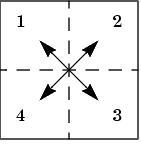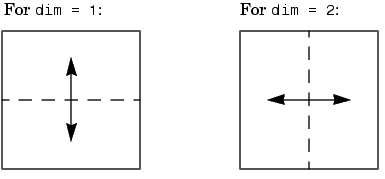MATLAB Function Referencefftshift

Shift zero-frequency component of discrete Fourier transform to center of spectrum

Syntax

• ```Y = fftshift(X)
Y = fftshift(X,dim)
```

Description

```Y = fftshift(X) ``` rearranges the outputs of `fft`, `fft2`, and `fftn` by moving the zero-frequency component to the center of the array. It is useful for visualizing a Fourier transform with the zero-frequency component in the middle of the spectrum.

For vectors, `fftshift(X)` swaps the left and right halves of `X`. For matrices, `fftshift(X)` swaps the first quadrant with the third and the second quadrant with the fourth.For higher-dimensional arrays, `fftshift(X)` swaps "half-spaces" of `X` along each dimension.

```Y = fftshift(X,dim) ``` applies the `fftshift` operation along the dimension `dim`.Examples

For any matrix `X`

• ```Y = fft2(X)
```

has `Y(1,1)` `=` `sum(sum(X))`; the zero-frequency component of the signal is in the upper-left corner of the two-dimensional FFT. For

• ```Z = fftshift(Y)
```

this zero-frequency component is near the center of the matrix.

See Also

`circshift`, `fft`, `fft2`, `fftn`, `ifftshift`

© 1994-2005 The MathWorks, Inc.